## Tuesday, December 31, 2013

### Installed wordpress on usb memory stick

Installed WordPress on a usb memory stick by making use of usbwebserver and part following guidelines from the following blog:
Carry WordPress in your Pocket : USB Webserver
So that may be I can experiment with custom themes, and otherwise building a website. At moment not sure I like having a blog as the main page to website, but then not sure what alternative to put there. Though I don't need a web server to test the alternatives: unless create a static home page in WordPress.

### FreeDOS and Ubuntu on Asus EEE PC Netbook

Accidentally killed MS Windows XP on my netbook. Used Partition Magic 8 to create partitions on my Netbook. All seemed to be going fine, then turned out that it wasn't. I got the netbook a while back now, the general idea was hopeful expectation that it would boot faster than Windows XP laptop, if it didn't then I would install MS DOS (Assuming I could figure out how to get around it being on 3.5" floppy disks) At the time I downloaded FreeDOS and Unbuntu for netbooks, but never got round to installing. Though I did burn CD's and otherwise load Ubuntu onto a bootable usb memory stick/flashdrive which was useful for fixing MS Windows problems. Also at the time I managed to network the netbook with my laptop share the laptop CD drive and install Powerquest Partition Magic on the netbook, though Bootmagic didn't seem to install. Any case problem was only allowed to have 4 primary drives and the netbook already had so I didn't pursue any further: primarily because have no Windows XP disks to re-install the system. On top of which at the time didn't fully understand how to get the system back without installation disks: it seemed like needed to install over Internet or something. Instructions didn't seem clear, and I didn't have time to pursue further.

So this time round I took a closer look at the partitions and identified one of them as the recovery partition for Windows XP, this was after I removed its hidden status with Partition Magic, and rebooted and could see in windows explorer. After which I discovered I could have probably browsed the files in Partition Magic. Any how I proceeded to remove the 4 primary drives and create a single primary partition and an extended partition. In the extended partition I then set up swap partition and ext2 partition for Linux. That all seemed to go fine. I then installed Ubuntu netbook edition from the usb drive. That all seemed to go fine too. The grub bootloader was automatically setup, and allowed booting Windows XP, Ubuntu, and also the Win XP recovery drive. However I didn't like the Ubuntu netbook edition, as none of the user guides I could find gave instructions which matched the Unity desktop it used. For that matter one guide discussed a gnome desktop.

I did also install both FreeDOS and Ubuntu netbook edition in virtual machines on my 64 bit laptop using Oracle Virtual Box. It seemed to go fine. For that matter Ubuntu in the virtual box made a connection to the Internet and spent an hour or more downloading and installing upgrades. I thought these upgrades would change the desktop to match the more recent user guides but it didn't. Installing Ubuntu 12? into virtual box didn't go so smoothly. But then virtual box wanted to update. After Virtual Box update, and allowing more ram for Ubuntu the installation, with power supply, and Internet connection, the install went more smoothly. I also tried the 64 bit version of Ubuntu, that wasn't compatible. So things went relatively ok in virtual box: only problem with respect to FreeDOS was how do I get files and other software into the virtual machine. That seemed somewhat complicated: further no real value to using FreeDOS inside a virtual box under MS Windows 7. The real objective was to boot my netbook fast and otherwise install Turbo C and Turbo Pascal along with maybe Paradox, and take a look at some of my old programs. {As there are some memory problems running at the Windows Command Prompt}.

The real objective a fast DOS box for writing and number crunching: don't need all the fancy graphics, my HP 28S calculator doesn't have such. Switch a calculator on get straight to crunching numbers, switch a word processor on get straight to typing. Switch a Windows computer on, get straight to no where: all that power and flexibility is good but its not always appropriate.

So creating another partition for FreeDOS on my netbook was the next task: that's where I hit problems. Partition magic would no longer start, complaining about drive assignment. I ran Ubuntu from the flashdrive again and went through the install, its partitioner indicated that none of the drive was allocated.

I then downloaded and installed GParted on a Live CD. I ran that but had problems, didn't seem to have any touch pad or keyboard control: touch pad could move cursor but couldn't execute any commands or select anything. After booting and trying a few times, eventually it worked with mouse plugged into usb port. It confirmed that none of the drive was allocated. Hunting down this problem, seemed that the partitions overlapped or something similar. So whilst XP and Ubuntu didn't seem to mind, and seemed to run ok, the disk partitioner didn't like. I ran a program called TestDisk, that seemed to identify the problem, and could apparently fix the problem. That is where I did something wrong or missed something: as after the fix I lost the bootloader and thus access to everything. One dead netbook.

Well not entirely dead as the data obviously still there on each partitions. So that using Ubuntu from a Live CD and further use of TestDisk could probably get everything back and adjust the start and finish of each partition. But seemed all too hard. So I just adjusted the partitions on the hard disk, created a partition for FreeDOS and installed FreeDOS then installed the most recent stable version of Ubuntu, which created a new bootloader menu for both FreeDOS and Ubuntu. So my netbook can now run either FreeDOS or Ubuntu.

Though the partitions are still not right. FreeDOS complains about faulty partition starting at 1 MByte, but when using GParted such no longer shows up: though it did when I created the partition. For some reason it had 1 Mbyte of free space before the partition. I simply accepted this. As another thing I discovered is that there should be unallocated space between a primary partition and a extended/logical partition: I didn't do this at the beginning. I did do this when I created new partitions. I don't remember doing such several years back when I set up my desktop for Windows XP, MS DOS and Linux.

Part one of objective achieved the netbook boots into FreeDOS fast. Only problem now is getting data to and from the netbook. It doesn't have any CD Drive, nor floppy disks, only usb: and DOS doesn't recognise USB drives without additional software. Problem is how to get that additional software onto the FreeDOS drive if it doesn't recognise the usb flashdrive. For installation can boot to Unbuntu, it recognises the usb drive and the FreeDOS drive, and can therefore copy files across. For general operations however booting to Ubuntu for copying files not practical when objective is a fast booting device. So hopefully can get the USB drivers to work in FreeDOS. If can then that would be good.

Already copied Turbo C and "As Easy As" over to FreeDOS drive. So far only tested that they open. As Easy As is a Lotus 123 like spreadsheet: a relatively small program and cost considerably less than Lotus, but was mostly compatible with: so something to crunch numbers with.

## Saturday, December 14, 2013

### Exporting Blogger Posts to individual html files

A few months back I exported my blogger blog, producing an atom xml file. I looked at this in XML notepad 2007 and it didn't seem very helpful. I also opened in MS Excel, from there at least I was able to extract a list of titles and keywords, but otherwise a bit messy. I had been experimenting writing own parser for my own simple xml files, the atom xml however seemed too complicated especially the element which contains the html formatted post.

I looked around for off-line editors, but none of the ones I could find seemed to be worthy of the title editor, as they are mostly for creation and posting of new posts, rather than editing and managing existing. What I wanted was to extract the individual posts and edit and possibly update the original posts or post again as a new post: noting that this blog is catharsis and basic brain dump of ideas.

Any case more recently I have been experimenting with the MSXML COM automation object, this I used in my simple barrier program to provide the data for the drop down list and associated load tables. So having had some success programming MSXML in both MS Excel/vba and then translating to vb.net, I thought I would take a look at ripping the blogger atom xml file apart with MSXML. {yeh! I'm now aware that I probably shouldn't use MSXML in vb.net}

Which turned out not to be all that complicated in the first instance. The xml file contains a lot of elements called <entry>, not all of these are posts. From looking at the file with XML notepad as far as I could tell those posts which have element <type> with value "html" are the posts and pages of the blog. The following code extracts the appropriate nodes.

Set nList = xmldoc.selectNodes("//entry[content/@type='html']")

Admittedly working out how to select the appropriate nodes wasn't so simple. Currently having problems understanding what I actually grab from the xml data, and what the text property is going to return, or value property returns.

Having got these nodes the next step was to find the child nodes with the required data and then write to either a plain text file or html file. For the initial test I just grabbed the data and wrote to a plain text file. Before writing the program I also copy/pasted the content node data using XML notepad and pasted to notepad and saved as simple text, renamed and viewed in google chrome. The whole xml file can also be viewed in Google chrome: writing a chrome application may be a alternative possibility for extracting the blog posts, but creating local files with a browser is slightly problematic
.
Once confirmed that could grab the posts, I added a few lines of additional code to create a more completely formatted individual html file. Only I was missing the post titles and getting those was more problematic. Well! initially I had everything for the <entry> element printed in the individual files, or otherwise just grabbed the content. So more research on XPath at w3chools.com, and more experimenting with select nodes, to arrive at the search string shown above.

With that search string have to process the child nodes of the node list to get to the <content> and <title> elements. Once I got the titles back into the posts, I figured I had a lot of files and I didn't know what they were as I merely gave them numbers. So I further modified the program to create an index file. So having got all that working on Friday, I figured the next step would be to do something with the categories or keywords assigned to each post. This posed another problem as not every <category> element contains a keyword, nor does it have a value, it only has attributes. Trying to find information on how to access the attributes wasn't easy.

Anycase there are two attributes scheme and term. It seems that "term" contains the keyword if the "scheme" attribute contains "http://www.blogger.com/atom/ns#". Therefore needed to be able to test the value of the attributes and extract the keywords.

Having figured out how to do this, I wrote the keywords to a table in Excel and counted the frequency of use in the blog posts. The code I used is as follows, it will need modifying to use elsewhere, but it my be useful as outline of basic process of getting information from the blogger atom xml.  Note that some of the code has wrapped to display into the width of the blog post, and the code needs to be on a single line.

```Sub xmlSimpleList2()
Dim wrkbk As Workbook
Dim wrkSht As Worksheet
Dim WrkRng As Range
Dim fpath1 As String
Dim keyWordTable As Range
Dim keyWord As String

Dim xmldoc As MSXML2.DOMDocument
Dim xmlNode As MSXML2.IXMLDOMNode

Dim tmpNode As MSXML2.IXMLDOMNode

Dim nList As MSXML2.IXMLDOMNodeList
Dim tmpList As MSXML2.IXMLDOMNodeList

Dim oNode As MSXML2.IXMLDOMNode
Dim oAttributes As MSXML2.IXMLDOMNamedNodeMap

Dim tagName As String
Dim tagValue As String
Dim i As Integer, j As Integer
Dim fp As Integer, fp1 As Integer

Set wrkbk = ThisWorkbook
Set wrkSht = wrkbk.Worksheets("xmlData")
Set WrkRng = wrkSht.Range("A1:A1")
Set keyWordTable = ThisWorkbook.Worksheets("keyWords").Range("A1:A1") 'row zero is header

Set xmldoc = New MSXML2.DOMDocument

xmldoc.async = False
If xmldoc.Load(myDocName) Then
fp1 = fopen(ThisWorkbook.Path & "\BlogPosts\" & "index.htm", "wt")
Print #fp1, "<html>"
Print #fp1, "<body>"
Print #fp1, "<ol>"

'Test XPath
i = 1
j = 1
' Set nList = xmldoc.selectNodes("//content[@type='html']")
Set nList = xmldoc.selectNodes("//entry[content/@type='html']")

' Final text entity is part of the tree, but attributes are not
For Each oNode In nList
tagName = oNode.nodeName
' Debug.Print i, tagName
WrkRng.Offset(i, 0).Value = tagName

fpath1 = ThisWorkbook.Path & "\BlogPosts\" & "post" & StrLPadc(CStr(i), 4, "0") & ".htm"
fp = fopen(fpath1, "wt")
Print #fp, "<html>"
Print #fp, "<body>"

If oNode.hasChildNodes Then
' Debug.Print "Number of child Nodes: " & oNode.childNodes.Length
For Each xmlNode In oNode.childNodes

Select Case UCase(xmlNode.nodeName)
Case UCase("title")
Debug.Print xmlNode.Text
WrkRng.Offset(i, 1).Value = xmlNode.Text
Print #fp, "<h1>"
Print #fp, xmlNode.Text
Print #fp, "</h1>"

Print #fp1, "<li><a href=" & """" & fpath1 & """" & ">" & xmlNode.Text & "</a></li>"

Case UCase("content")
Debug.Print "content"
Print #fp, xmlNode.Text

Case UCase("category")
Debug.Print "category"
Set oAttributes = xmlNode.Attributes
Set tmpNode = oAttributes.getNamedItem("scheme")

If tmpNode.Text = bloggerNameSpace Then
j = j + 1
Set tmpNode = oAttributes.getNamedItem("term")
keyWord = tmpNode.Text
WrkRng.Offset(i, j).Value = keyWord
Call updateKeyWordList(keyWordTable, keyWord)
End If

End Select

Next xmlNode
End If

Print #fp, "</body>"
Print #fp, "</html>"
Close #fp
i = i + 1
j = 1
Next oNode

Print #fp1, "</ol>"
Print #fp1, "</body>"
Print #fp1, "</html>"
Close #fp1

End If  'xml doc loaded

Set xmldoc = Nothing

Debug.Print "All Done!"

End Sub
```

## Friday, December 06, 2013

### Specifications Governing Structural Engineering Software Used by Non-Engineers

There are two documents controlling software for "structural engineering" software to be used by persons who are not structural engineer's these are:

1. South Australian Minsters Specification SA A2.2 Structural Engineering Software (2010)
2. Australian Building Codes Board (ABCB) : Protocol for Structural Software version 2011.1
Both documents seem to impose a lot of paper shuffling nonsense into the exercise, and seriously miss the point and purpose of much of the software. The software will ultimately be used by customers the end-users of the structures, currently the software is used largely by sales people. To consider training and certifying the customer, the ultimate end-user of the structure being checked is silly. The whole point of the software is to be based on input parameters which are within the comprehension and interests of the end-user, and the outputs of the software are equally to match the end-users interests.

The software has to translate the end-users needs into technical requirements compliant with codes of practice behind the scenes. Code requirements and constraints are not something the end-user needs to deal with nor are they interested in.

The regulatory wants and whims have to be dealt with separately. It is not software that needs to be dealt with, it is repetitively manufactured structural products which need to be properly specified which needs to be dealt with. These structural products can be parametrically varied with in limitations, however I contend it is not acceptable for the specification to be buried within software simply because the software can deal with the variations. A written specification is still required and it will form the basis of the software.

It is really annoying when a manufacturer wants engineering input for a variation beyond the limits of their software, and they have no information what so ever about their product other than a black box computer program they push numbers through. It then becomes necessary to experiment with the black box to see if can gleam any idea of how it makes its assessment, and whether or not agree with it. At least when the suppliers held standard calculations, could look at the calc's and quickly conclude the assessment was inadequate or  otherwise acceptable.

As one major problem with structural products is most consultants have no idea how the product can work. So they do calculations and conclude product not acceptable in the first place, so no way they can prove for the variation the manufacturer wants. One part of the problem is that standard products are based on testing, the other part of the problem however is that the original assessment by calculation is just plain incomplete. Now that incomplete assessment is getting hidden away and buried in proprietary software only available to manufacturers and their agents: not available to people who are responsible for granting regulatory approval to go ahead and build.

I don't however believe the solution to the problem involves generating large quantities of paperwork and signatures. The building industry just has not grasped QA versus QC. Quality cannot be inspected in, it has to be designed in. Similarly safety cannot be inspected in, it also has to be designed in. Design of the process doesn't involve creating a system which collects lots of signatures. Also a QA system accredited by a third party, is actually a QC system. If understand QA, then you do not have an accept or reject mentality, therefore getting accreditation or not by some supposed QA authority is contrary to QA philosophy.

Unfortunately the building regulator thought the idea was to get rid of inspections, and opened the door for non-compliance with approved documents. They have now put much of the inspection back, but they still missed the point. QC inspects at the end, and rejects the construction: and thus wastes time and materials. QA makes sure they get it right in the first place, and that requires an entirely different approach.

Quality considered in terms of signal to noise ratio (S/N), the building industry is still focused on boosting the signal rather than filtering out the noise. Hence lots of paperwork generated.

Training people to do the work, is somewhat Procrustean, as it takes the approach of make the person fit the job, rather than design the job to fit the people. The software is meant to be for untrained people, therefore it has to be designed accordingly. So training users, getting them certified, maintaining registers, and keeping certifications up to date, is just plain nonsense. As an interim measure whilst the software is not appropriately designed for the skills of the actual end-users, then training may be necessary. But it shouldn't be a requirement.

Also the requirement that the software be authored by a structural engineer is unacceptable. For certain a structural engineer needs to contribute to the software, but they have far inadequate training to design and develop appropriate user interfaces and error checking systems for software. The structural engineers are partly responsible for the software being rubbish and giving rise to the two controlling documents. The structural engineers may have got the structural calculations behind the scenes right, but the software itself is poor in terms of its user interface's: both the input and the output.

No one seems to be paying all that much attention to the point and purpose of calculations or engineering. Its all reduced to : will you sign off on this, will you provide a certificate. The fundamental basis of our system is adequate evidence-of-suitability, and a mere signature cannot be defended during a coroners inquest.

The two controlling documents provide a good starting point and guidance, but some of the requirements are highly obstructive are going to cause a lot of problems, and are unnecessary.

## Wednesday, December 04, 2013

### On Developing Structural Analysis 2D Plane Frame Application

Back when we started, we didn't have any frame analysis software. First problem was knowing what was available and where to get it from: it wasn't like could just go down the street and buy from local computer store. Second problem was such software was too expensive.

Our business doesn't work on debt: taking out loans and hoping will get the business to repay the loan is risky: possibly crazy. Since we had time we invested time: a kind of sweat equity. Whilst hoping for a return on the investment of time,  there is no requirement financially, as there is no real debt. Sure economists may throw opportunity costs in the mix and say there is debt. That is it would be considered better to buy the tools and put them to use and start to make money from using the tools rather than expend time creating the tools. The answer to that however is that the only tools required are pencil/paper and a calculator. If can do the work starting with blank piece of paper, then possibly also able to start with a blank computer screen, if can program. Sure the tools develop oneself may not be as good as a bought one. Then again the bought ones may lack certain features. If buy software then constrained to what it does and the way it does it, and also have to wait for authors of the software to update the software to new codes of practice. Then there is a problem of whether the software remains in the market. One consultant had advantage in the cold-formed steel market because he had frame analysis software which designed to AS1538 permissible stress version of cold-formed steel code. Problem was, the company ceased to operate and the software was never updated to AS4600. The consultant had advantage because of what others could do, not because of what he could do.

My philosophy has always been we build those tools ourselves which are practical to build and directly related to what we do: the core activity of engineering design. Not going to waste time building massive complex integrated tools. However with the passage of time the tools which become practical to build in-house increases. It is all dependent on heritage, and building foundations for future development.

So we were only dealing with plane frames. My dad could analyse using moment distribution, I could have learnt such, but speed wise better to use Kleinlogel formula available in steel designers manual {I'm assuming current version has same as earlier versions. Also being mechanical my formal studies didn't go in depth into the analysis of rigid frames.}. So all up we didn't really need frame analysis software, but it would have made things easier and faster.

We had the book: Microcomputer Applications in Structural Engineering by W.H Mosley and W.J Spencer. This book contained several programs written in Basic. I had typed them all into the computer, whilst I was still studying. My dad took the plane frame program and translated into Turbo Pascal, and turned from a cumbersome command line program to one with a graphical interface. Whilst I was experimenting with Turbo C and menu systems, my dad experimented with Turbo Pascal. I opted for C as a programming language, as it was apparently to be the future for AutoCAD. My dad opted for Pascal because it was the language being used on the graduate diploma in computer science he studied. I did learn Pascal as, Turbo Pascal was the first compiler we got for the CPM/80 machine. It wasn't until a few years later when got an MS DOS machine that I got Turbo C.

At this point not releasing the program, as it has our business details hard coded into the reports. It is also proving difficult to recompile a MS DOS program in the windows command prompt as having memory problems. Something to do with the command prompt environment I'm guessing. Also the program doesn't run on my Windows 7 computer: though very little does as its 64 bit: its good at collecting dust. A few years back my dad did convert into vba to run in Excel, and about 2 years back I wrapped it into a vba class. But still looking to get it back into a stand alone application, possibly even a COM automation object, or what ever the equivalent for .net is. Unfortunately the benefits of adopting vb.net to take advantage of all the Excel/vba code I have written are not that apparent: as vb.net has some major differences compared to vba. Therefore looking at going back to Pascal, at the moment managed to rip out all the Turbo gadget graphics and create a console application using Borland Delphi. Given its no longer Borland product, considering adopting Lazarus and free Pascal. The problem I have with that approach is having to revise all my Delphi wind loading procedures from AS1170.2:1989 to AS1170.2:2011, or otherwise translate Excel/vba into Pascal. So may look at parallel developments to integrate everything together.

My current Excel/vba workbooks are using Kleinlogel formula in the worksheets and therefore restricted in the frame they can quickly assess. To change the frame more work required in the worksheet, and that makes it cumbersome to change from one frame to another. Having the frame analysis in vba behind the scenes still doesn't really improve the situation. It is currently being used for carport/canopy design in that manner for one carport supplier: that however has limited capabilities and a small amount of my code in it. Another workbook I have set up using more code and dialogue boxes keeps hitting Excel/vba memory limits. Therefore need to get the code out of Excel/vba. Also having worksheet formula creating a 20Mbyte Excel workbook and needing MS Excel to use that workbook, so as to do what a stand alone 250Kbyte program can do, is really inefficient use of computing resources. The industry keeps running around seeking spreadsheets, because they think that is the simple approach: but then they want greater flexibility in design options than is practical to implement by spreadsheet, and which is also kept within the capabilities of any salesperson.

Spreadsheets and in worksheet formulae are practical for all the dimension and load calculation's prior to using frame analysis software. Spreadsheets and worksheet formulae also practical for design members and connections after frame analysis. But frame analysis in the worksheet formulae is only practical for simple structures: like beams and very simple frames.

That's where the bottleneck emerges. Very simple structural analysis and design, can be completed quickly from a few parameters input to a spreadsheet. Increase the complexity and the integration is loss. Very little frame analysis software has facility to automatically generate the model: a full model that is. Most frame analysis software can generate dimension and geometry for some common structural forms. But the software doesn't calculate and assign the loads to the structural elements. The commercial frame analysis software can also check/design the members once the analysis is complete: depending on materials. Cold-formed steel is not a material commonly available as a design module with commercial software. Therefore have to check members independently, this posing another delay. Then there is the design of connections which also has to be external to the analysis software. The use of BIM can maybe streamline this process for custom buildings, but BIM is typically does not provide parametric modelling of common structural forms.

Just as Toyota aimed for single minute exchange of dies, to improve manufacturing productivity: design and engineering suppliers need also to be looking at single minute design, assessment and documentation, and for that matter even approval. That is, concept to approval in one minute. Not for all structures and buildings, just those which have common structural forms, and are extremely routine. Unfortunately the nail plated timber roof industry use of software through a spanner in the works. It is therefore necessary to avoid those problems, and that requires software which is not proprietary and locked to manufacturers of structures: at least some version of the software needs to available to the whole supply chain: which includes the certifying authorities. Any case I will ramble on more, about that at a later date.

For now here are some screen shots of the application I am breaking apart and converting over to windows.Sample Data File viewed in UEStudioOpening ScreenMain MenuOpen Data File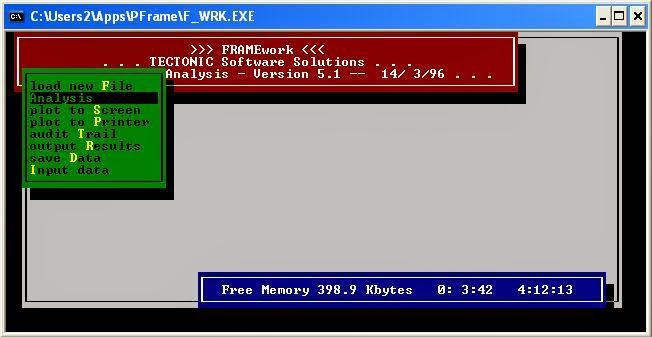AnalysisScreen Plot Menu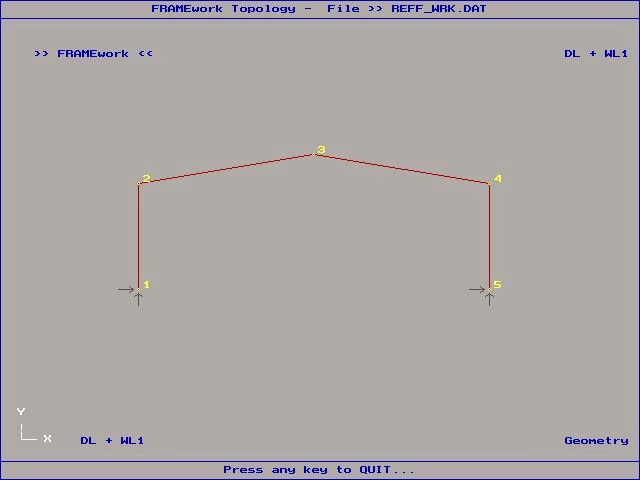Geometry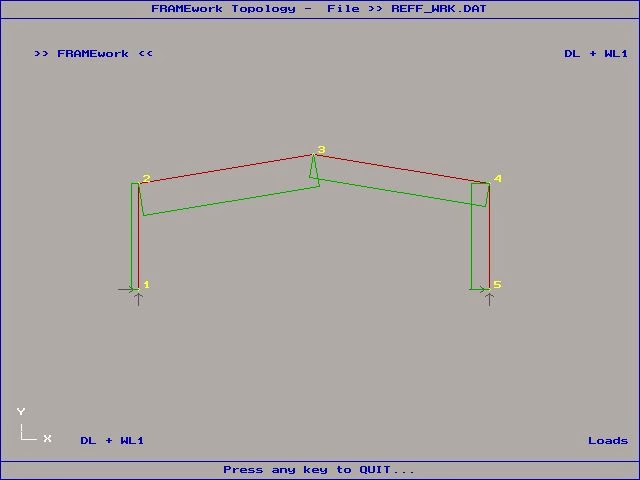Design ActionsAction-Effect: Bending MomentAction-Effect: ShearAction-Effect: Axial ForceAction-Effect: Exaggerated Deflections
Which is typically the way I used the program, as I generated the data file using Quattro Pro, rather than using the user interface. For me the application would have been better if it provided a command line option to read data file and produce results file without need to use user interface. When moved from Quattro Pro to MS Excel, lost some of the benefits of the original QPro application, though gained other benefits and moved in other direction. So part of current task is resurrecting the QPro application and finally converting over to Excel/vba.

Any case the plane frame application did allow creating and editing data files, even had facility to print the screen plots to the printer: though locked to a specific printer. The data input screens are as follows.Main Input MenuMain Menu to define structure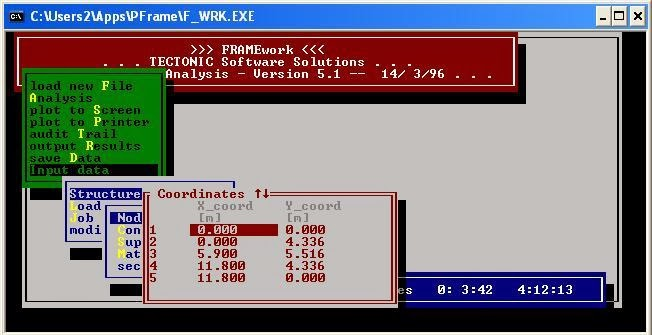Node DataMember ConnectivitySupportsMaterials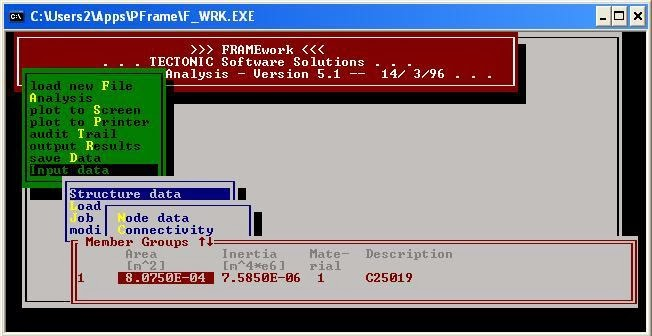Sections
If I recollect, there is an error in the unit description for the section properties. Not a problem if know what is required in the data file. But a good reason not to release the program.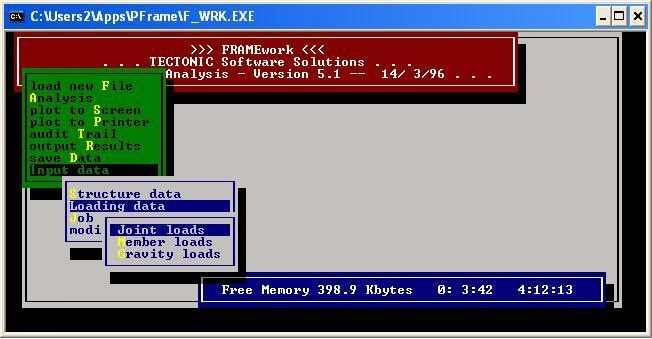Main Menu for LoadsJoint Loads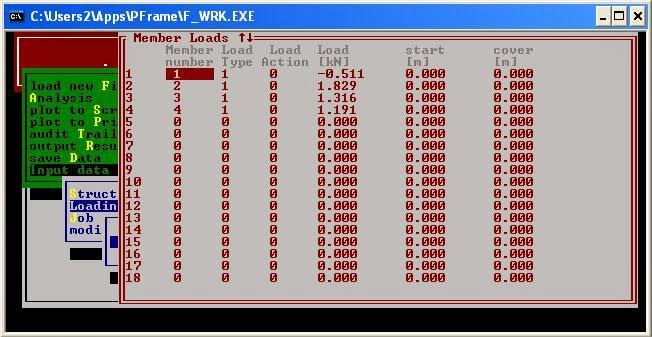Member Loads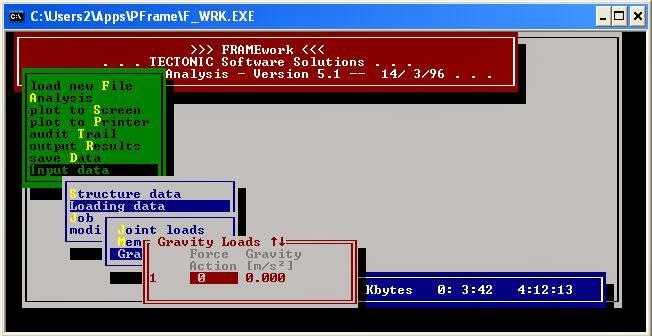Gravity Loads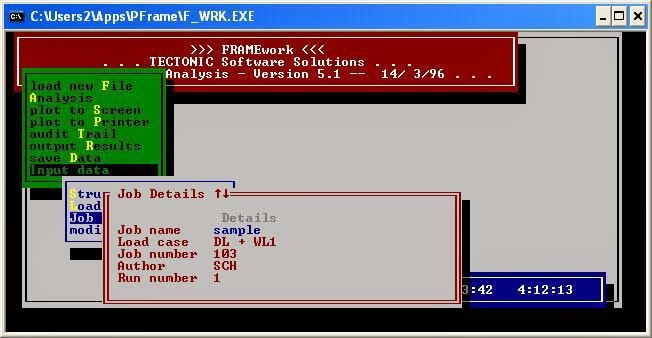Job Details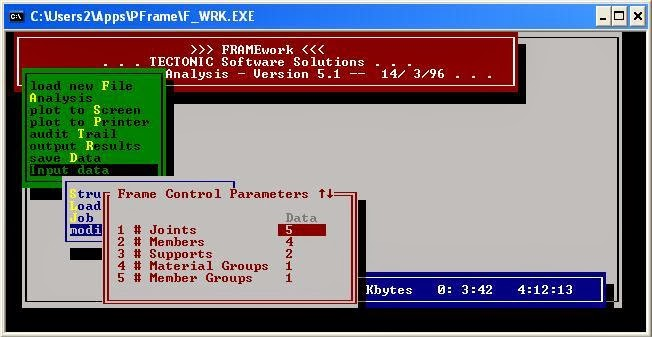Control Data
Whilst the control data is the last menu item, it needs to be input first. That is a cumbersome aspect of the program. Part of the conversion from the original Basic source. The original program asked questions on the DOS command line, if got an input wrong then couldn't back track, had to cancel and start again. Part of the requirement being to set up the application environment.

Our Pascal version of the program however has dynamically allocated variables, and makes use of record structures and pointers. I think it also makes use of sparse matrices as well. It therefore should not be necessary to input the control data, as it should be able to work it out. Such data should thus also not be required in the data file. So rewriting the file input routines is another part of the current exercise, otherwise creating a new file format, possibly using XML, and a file translator for the older files.

So at the moment looks like parallel developments in Pascal, vb.net and Excel/vba, with possible experimenting with Java. When reach the point of having either a DLL or COM automation library, then should all converge and move over to a single programming environment. Each programming language has its own unique library of built-in functions which become an obstacle when move over to an another language and such functions are not available. It represents more programming before can move forward. Basically each programming language has a different heritage, and therefore different foundations on which to build.

So attempting to bring a collection of tools, developed on as needs basis, together as an integrated whole. Which they were a lot closer to being at the very beginning when we started, but for various reasons they diverged.

Sample Output:Results File: Showing Definition of Structure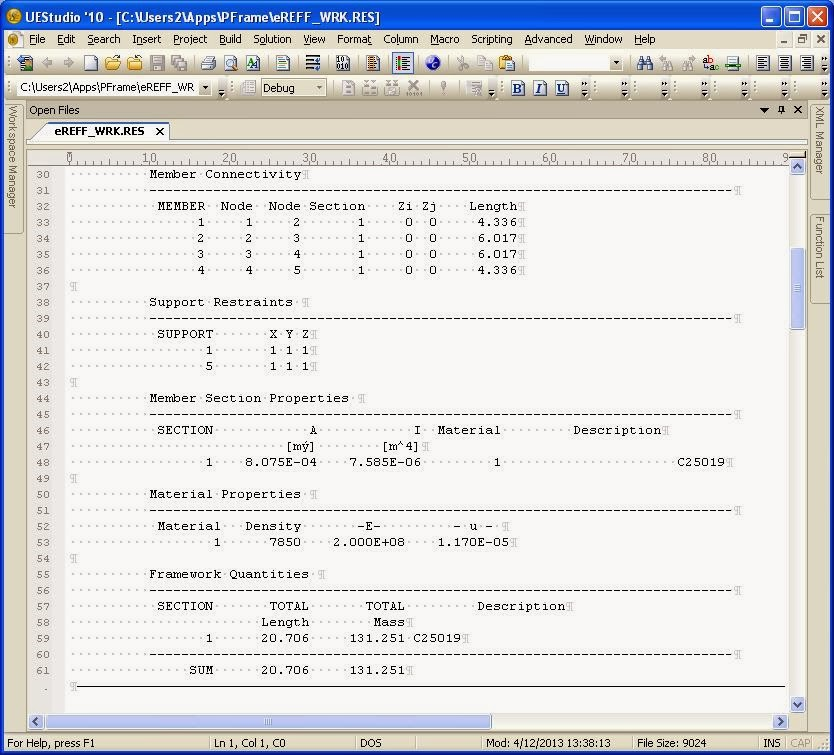Results File: More definition of Structure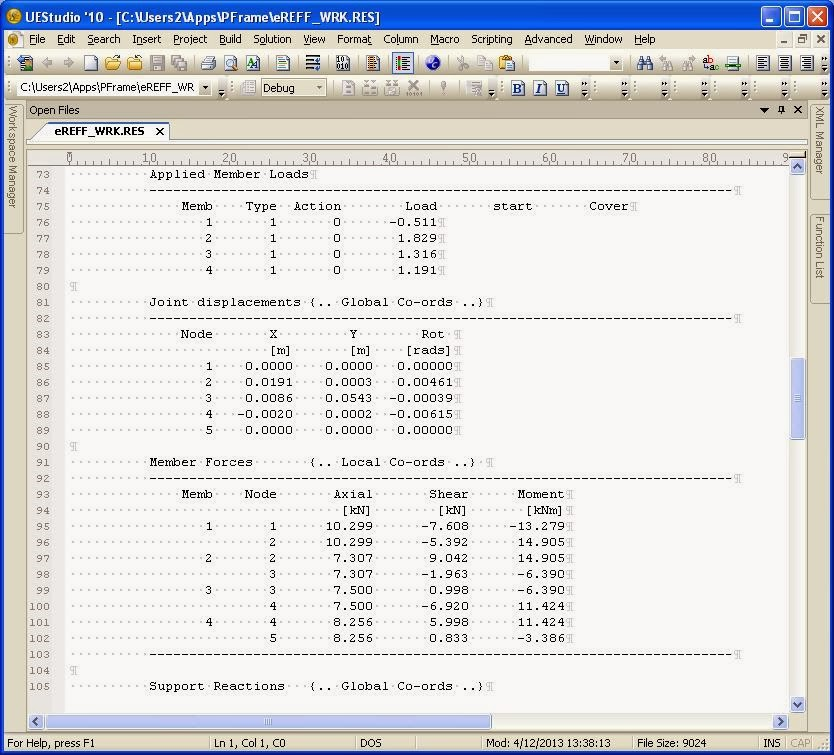Results File: Applied Loads and Calculated Member Forces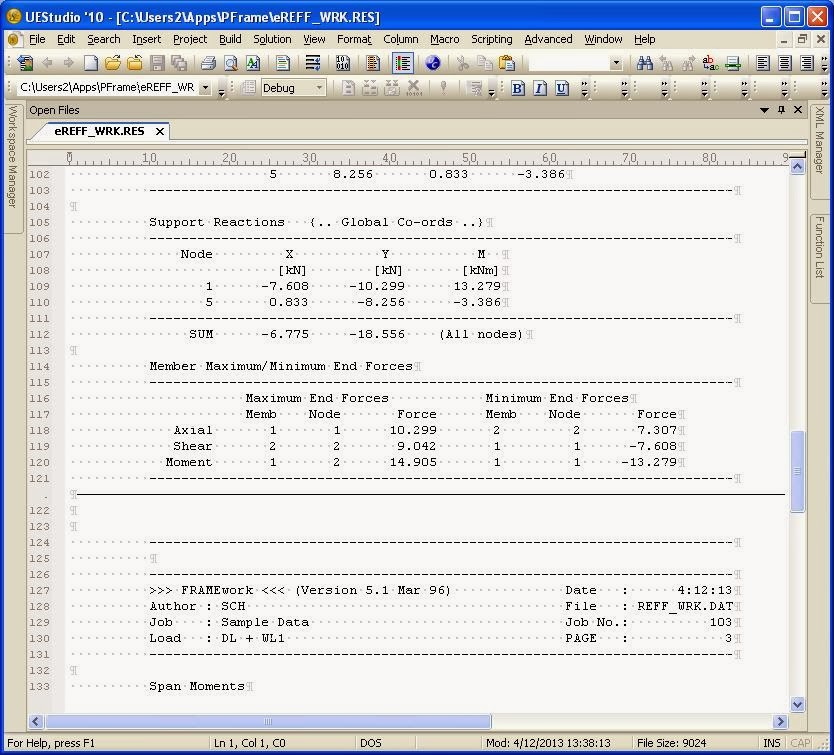Results File: Support Reactions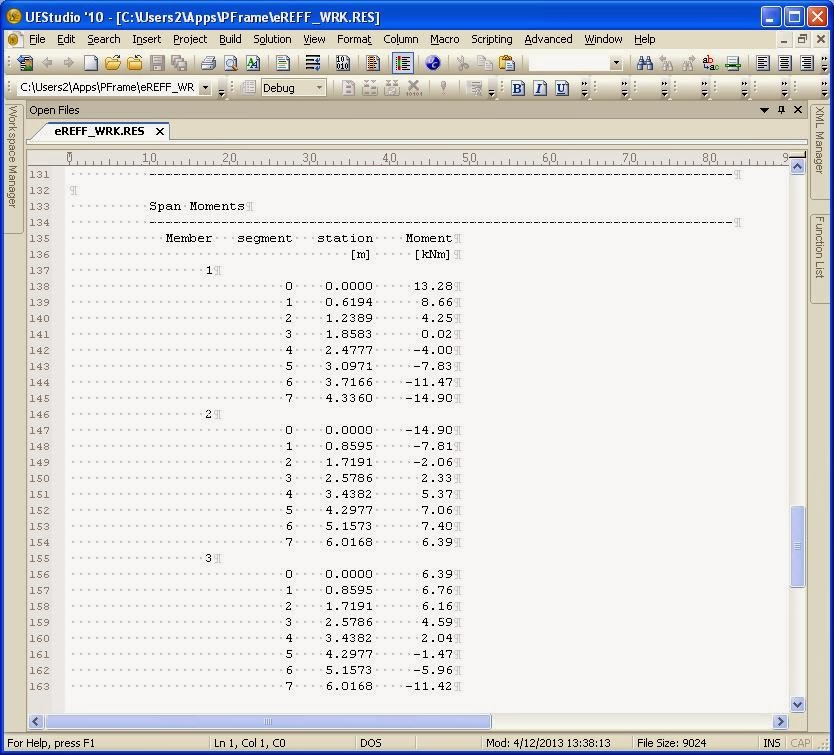Results File: Moments in Span of Each Member part 1Results File: Moments in Span of Each Member part 2

Related Posts:

Download Page for The Plane Frame Analysis Application

## Tuesday, December 03, 2013

### schTechLIB contents

A rough listing of Functions and Subroutines contained in the schTechLIB. The modules were all extracted using Excel vba CodeCleaner by AppsPro. Then FileLocator Pro regular expressions were used to find the functions and subroutines and report. Not all the parameters are listed as some function/subroutine headers span multiple lines, also some data was lost when the reports were imported into MS Excel and sorted. But its a start at documenting the content.

 Buildings Building000.bas Function IsCanopyStructure(StructuralForm As Integer) As Boolean IsEnclosedBuildingStructure(StructuralForm As Integer) As Boolean IsRoof(BuildingSurface As Integer) As Boolean IsWall(BuildingSurface As Integer) As Boolean IsWindWard(BuildingSurface As Integer, theta As Double) As Boolean SurfaceStr(Surface As Integer) As String Building001.bas Function IsAttachedCanopy(StructType As String) As Boolean IsCanopy(StructType As String) As Boolean IsDomestic(StructClass As String) As Boolean IsGable(RoofType As String) As Boolean IsHipped(RoofType As String) As Boolean IsIndustrial(StructClass As String) As Boolean IsRural(StructClass As String) As Boolean IsShed(StructType As String) As Boolean IsSkillion(RoofType As String) As Boolean IsTroughed(RoofType As String) As Boolean Design Actions LimitStateConstants.bas Sub SetLimitStateTables() LoadActions001.bas Function CalcLiveLoad(a As Double) As Double MagnifyLoad(a As Double, k1 As Double, k2 As Double, k3 As Double, k4 As Double) As Double StructuralLoading001.bas Function DistributedLoad(AreaLoad As Double, loadwidth As Double) As Double TotalLoad(AreaLoad As Double, _ StructureLoads.bas Sub TStructureLoads_Init(xLoad As TStructureLoads) Files fmFileMngt.bas Function FileExists(SearchSpec As String) As Boolean FirstFileFound(SearchSpec As String, fattr As Integer, foundname As String) As Boolean NextFileFound(foundname As String) As Boolean fmOpenFilesTEXT.bas Function fopen(fname As String, fmode As String) As Integer Sub fopenTXT(fp As Integer, fname As String, fmode As String) Geometry Geom3D03.bas Sub SetCoord(pt As TCoord, x1 As Double, y1 As Double, z1 As Double) WriteCoord(fp As Integer, pt As TCoord) Geom3D05.bas Sub SetDescr(node As TNode, descr1 As String) SetNode(node As TNode, descr1 As String, x1 As Double, y1 As Double, WriteNODE(fp As Integer, node As TNode) Geom3D06.bas Sub SetEdge(n1x As Integer, n2x As Integer) WriteEdge(fp As Integer) Mathematics xMathsAreaProperty.bas Function CircleArea(d As Double) As Double RectangleArea(b As Double, h As Double) As Double TriangleArea(b As Double, h As Double) As Double xMathsExponentials.bas Function Log10(x As Double) As Double LogN(x As Double, n As Double) As Double Power(a As Double, x As Double) As Double xMathsInterpolation.bas Function Calc_X(x1 As Double, y1 As Double, x2 As Double, y2 As Double, y As Double) As Double Calc_Y(x1 As Double, y1 As Double, x2 As Double, y2 As Double, x As Double) As Double Slope_M(x1 As Double, y1 As Double, x2 As Double, y2 As Double) As Double YIntercept_C(x1 As Double, y1 As Double, x2 As Double, y2 As Double) As Double xMathsNumbers.bas Function Ceil(x As Double) As Double Floor(x As Double) As Double Frac(x As Double) As Double MaxValue(Recrds() As Double) MinValue(Recrds() As Double) Sub dec(ByRef i As Integer) inc(ByRef i As Integer) MathTest1() xMathsRightTriangle.bas Function Polar_Angle(x As Double, y As Double) As Double Polar_Radius(x As Double, y As Double) As Double RectCoord_x(r As Double, theta As Double) As Double RectCoord_y(r As Double, theta As Double) As Double RightTrig_Adj(Hyp_r As Double, Opp_y As Double) As Double RightTrig_Cos(Hyp_r As Double, Adj_x As Double) As Double RightTrig_Hyp(Adj_x As Double, Opp_y As Double) As Double RightTrig_Opp(Hyp_r As Double, Adj_x As Double) As Double RightTrig_Rise(Adj_x_Run As Double, theta As Double) As Double RightTrig_Run(Opp_y_Rise As Double, theta As Double) As Double RightTrig_Sin(Hyp_r As Double, Opp_y As Double) As Double RightTrig_Tan(Adj_x As Double, Opp_y As Double) As Double xMathsSortingSubs.bas Sub Sort(Recrds() As Double, Index() As Integer, n As Integer) SwapDouble(Arg1 As Double, Arg2 As Double) SwapInt(Arg1 As Integer, Arg2 As Integer) xMathsTrigonometry.bas Function ArcCos(x As Double) As Double ArcSin(x As Double) As Double From_HMS(h As Double, m As Double, s As Double) As Double From_HMS2(theta As Double) As Double get1stQuadAngle(theta As Double) As Double getRationalAngle(theta As Double) As Double pi() As Double To_HMS(theta As Double) As String To_HMS2(theta As Double) As String ToDegrees(x As Double) As Double ToRadians(x As Double) As Double Mechanics BeamBM.bas Function BeamBM1(BM1 As Double, r1 As Double, w As Double, x As Double) BeamCantilevers.bas Function BM_Cant1(w As Double, x As Double, L As Double) As Double BeamFF1.bas Function BM_FF1(w As Double, x As Double, L As Double) As Double def_FF1(w As Double, x As Double, L As Double, EI As Double) As Double defMax_FF1(w As Double, L As Double, EI As Double) As Double FEM_FF1(w As Double, L As Double) As Double Ra_FF1(w As Double, L As Double) As Double Rb_FF1(w As Double, L As Double) As Double BeamFF2.bas Function BM_FF2(w As Double, x As Double, a As Double, b As Double, L As Double) As Double FF2_Ra(w As Double, a As Double, b As Double, L As Double) As Double FF2_Rb(w As Double, a As Double, b As Double, L As Double) As Double Sub TestFF2() BeamOverHang.bas Function BM_Ohang(P As Double, x As Double, a As Double, b As Double) As Double def_Ohang(P As Double, x1 As Double, a As Double, b As Double, EI As Double) As Double BeamSS.bas Function eq_udl1(n As Integer, w() As Double, a() As Double, _ SS_Beam_BM(w As Double, L As Double, _ SS_Beam_BM2(w As Double, L As Double, _ SS_Beam_RA(w As Double, L As Double, _ SS_Beam_RA2(w As Double, L As Double, _ SS_Beam_RB(w As Double, L As Double, _ Sub Beam3() BeamSS1.bas Function BM_ss1(w As Double, x As Double, L As Double) As Double def_ss1(w As Double, x As Double, L As Double, EI As Double) As Double defMax_SS1(w As Double, L As Double, EI As Double) As Double Ra_SS1(w As Double, L As Double) As Double udl_ss1(BM As Double, L As Double) As Double BeamSS2.bas Function BM_ss2(w As Double, x As Double, a As Double, b As Double, L As Double) As Double BM_ss2c(w As Double, x As Double, a As Double, b As Double, c As Double) As Double defMax_ss2(w As Double, _ R_ss2(w As Double, a As Double, b As Double, L As Double, ReactionNode As String) As Double Sub ss2(w As Double, a As Double, b As Double, L As Double, _ ss2c(w As Double, a As Double, b As Double, c As Double, _ BeamSS3.bas Function BM_ss3(w As Double, x As Double, L As Double, a As Double) As Double def_ss3(w As Double, x As Double, a As Double, L As Double, EI As Double) As Double Ra_SS3(w As Double, L As Double, a As Double) As Double Rb_SS3(w As Double, L As Double, a As Double) As Double Sub ss3(w As Double, L As Double, a As Double, _ BeamSS9.bas Function BM_ss9(P As Double, x As Double, a As Double, L As Double) As Double def_ss9(P As Double, x As Double, a As Double, L As Double, EI As Double) As Double R_ss9(P As Double, a As Double, b As Double, L As Double, ReactionNode As String) As Double BoltProp.bas Function AS4100_BoltShear(fuf As Double, kr As Double, _ AS4100_BoltTension(fuf As Double, A_s As Double) As Double AS4100_BoltTension2(d As Double, P As Double, fuf As Double) As Double MeanDiam(d As Double, P As Double) As Double MeanDiam2(d As Double, P As Double) As Double MinorDiam(d As Double, P As Double) As Double PitchDiam(d As Double, P As Double) As Double TensileArea(d As Double, P As Double) As Double ENVMySystem.bas Sub SetMachine(ByRef fpathMtrl As String) Mechanics1.bas Function getMomentCapacity(f As Double, z As Double) As Double getRequiredSectionModulus(f As Double, BM As Double) As Double RadiusGyration(i As Double, Area As Double) SimpleStress(Force As Double, Area As Double) As Double MechDesign010.bas Function DesignResistance(phi As Double, Kmod As Double, fo As Double, GeomSectProp As Double) As Double FlexuralResistance(phi As Double, Kmod As Double, fy As Double, Ze As Double) As Double KResultant(k() As Double) As Double TensileResistance(phi As Double, Kmod As Double, fy As Double, Area As Double) As Double Sub TestMechDesign1() MechDesign090.bas Function getFx(f As Double, theta As Double) As Double getFy(f As Double, theta As Double) As Double Resolve_Fx(Axial As Double, Shear As Double, beta As Double) As Double Resolve_Fy(Axial As Double, Shear As Double, beta As Double) As Double ProppedCantilevers.bas Function BM_PC1(w As Double, x As Double, L As Double) As Double SectionProperties.bas Function Ixx_plate(b As Double, h As Double) As Double Steel SteelSection5.bas Function AS4100_get_bfo(Tf As Double, fy As Double) As Double AS4100_get_Zc(z As Double, s As Double) As Double AS4100_get_Ze(z As Double, s As Double, _ AS4100_get_Zn(z As Double, s As Double, lambda_s As Double, _ AS4100_get_Zs(z As Double, s As Double, _ AS4100_getAlpha_d(dp As Double, fy As Double, s As Double, _ AS4100_getAlpha_f(bfo As Double, Tf As Double, tw As Double, d1 As Double) As Double AS4100_getAlpha_m(Mmax As Double, m2 As Double, M3 As Double, M4 As Double) As Double AS4100_getAlpha_s(Ms As Double, Moa As Double) As Double AS4100_getAlpha_v(tw As Double, dp As Double, fy As Double, s As Double, _ AS4100_getLambda_e(b As Double, t As Double, fy As Double, isCHS As Boolean) As Double AS4100_getLambda_s(lambda_e1 As Double, lambda_e2 As Double, ByRef elem As Integer) As Double AS4100_getLe(kt As Double, kl As Double, kr As Double, L As Double) As Double AS4100_getMo(E As Double, G As Double, Iy As Double, J As Double, _ AS4100_getMs(fy As Double, Ze As Double) As Double AS4100_getphiMb(phi As Double, alpha_m As Double, alpha_s As Double, Ms As Double) As Double AS4100_getphiMs(phi As Double, fy As Double, Ze As Double) As Double AS4100_getphiVv(phi As Double, Vv As Double) As Double AS4100_getUnSymMo(E As Double, G As Double, Iy As Double, J As Double, _ AS4100_getVvm(Vv As Double, m As Double, phiMb As Double) As Double SteelCFAllSections.bas Function get_Ae_AS4600(d As Double, Bf As Double, t As Double, _ get_Zex_AS4600(d As Double, Bf As Double, t As Double, _ SteelCFBoxSectionProp.bas Function get_BOX_Ae(d As Double, Bf As Double, t As Double, _ get_BOX_J(a As Double, b As Double, t1 As Double, t2 As Double) As Double get_BOX_Zex(d As Double, Bf As Double, t As Double, _ Sub BOX_checkWeb(d As Double, d1 As Double, ri As Double, t As Double, _ BOX_CompressionFlange(Bf As Double, ri As Double, t As Double, _ BOX_CornerFillet(d As Double, ri As Double, t As Double) BOX_FullyEffective_Web(d As Double, ri As Double, t As Double, d1 As BOX_TensionFlange(Bf As Double, d As Double, ri As Double, t As Doub BOX_test() BOXSection_Prop(d As Double, Bf As Double, t As Double, _ Calc_BOX_Zex(ns As Integer, t As Double, ycg As Double, Ixx As Doubl SteelCFCSectionProp.bas Function get_Ae(d As Double, Bf As Double, t As Double, _ get_Zex(d As Double, Bf As Double, t As Double, _ Sub AS4100_getVw(fy As Double, Ae As Double, isCHS As Boolean) As Double AS4100_HasFullLateralRestraint(L As Double, Beta_m As Double, _ GetSlenderElement(lambda_e1 As Double, lambda_e2 As Double) As Integer IsCompact(lambda_s As Double, lambda_sp As Double) As Boolean IsNonCompact(lambda_s As Double, lambda_sp As Double, lambda_sy) As Boolean IsSlender(lambda_s As Double, lambda_sy) As Boolean IsTransverseStiffenedWebOk(t As Double, s As Double, d1 As Double, fy As Double) As Boolean IsUnstiffenedWebOk(t As Double, d1 As Double, fy As Double) As Boolean SteelSection6.bas Function AS4100_get_be(b As Double, lambda_ey As Double, lambda_e As Double, ElementType As Integer) As Double AS4100_get_kf(Ae As Double, Ag As Double) As Double AS4100_get_lambda_e(b As Double, t As Double, fy As Double, ElementType As Integer) As Double AS4100_get_Le(ke As Double, L As Double) As Double AS4100_get_Nc(alpha_c As Double, ns As Double) AS4100_get_Ns(kf As Double, An As Double, fy As Double) SteelSection633.bas Function AS4100_get_Alpha_a(lambda_n As Double) As Double AS4100_get_Alpha_c(xi As Double, lambda As Double) As Double AS4100_get_eta(lambda As Double) As Double AS4100_get_lambda(lambda_n As Double, alpha_a As Double, alpha_b As Double) As Double AS4100_get_lambda_n(le As Double, r As Double, kf As Double, fy As Double) As Double AS4100_get_xi(lambda As Double, eta As Double) As Double SteelSection7AxialTension.bas Function AS4100_getNt(Ag As Double, An As Double, _ SteelStructSections.bas Function ElementEffectiveWidth(SectionType As Integer, b As Double, _ getElasticityLimit(PlateElementType As Integer, _ getNumberOfEdgesSupported(SectElement As Integer, _ getPlasticityLimit(PlateElementType As Integer, _ getPlateElementType(SectionType As Integer) As Integer getSectionDescrCode(code As Integer) As String getSectionType(section As String) getStressGradientState(SectElement As Integer, SectionType As Integer, axis As String) As Integer isSectionCHS(section As String) As Boolean isSectionRHS(section As String) As Boolean isSectionSHS(section As String) As Boolean isSectionUB(section As String) As Boolean isSectionUC(section As String) As Boolean Sub LoadSectionCodes() Strings xstring.bas Function FmtAcadPath(fPath As String) As String fnDir(fPath As String) As String fnDrv(fPath As String) As String fnExt(fPath As String) As String fnmerge(fPath As String, drv As String, fdir As String, fname As String, fext As String) As String fnName(fPath As String) As String fnName2(fPath As String) As String strchr(s As String, c As String) As Integer StrLen(s As String) As Integer StrLPad(s As String, lStr As Integer) As String StrLPadc(s As String, lStr As Integer, c As String) As String strlwr(s As String) As String StrNset(s As String, ch As String, n As Integer) As String StrRChr(s As String, c As String) As Integer StrRPad(s As String, lStr As Integer) As String StrRPadc(s As String, lStr As Integer, c As String) As String strupr(s As String) As String substr(s As String, sp As Integer, L As Integer) As String Sub fnsplit(fPath As String, drv As String, fdir As String, fname As Str fnsplit2(fPath As String, drv As String, fdir As String, fname As St xStrLib.bas Function isAlnum(ch As String) As Boolean isAlpha(ch As String) As Boolean isCntrl(ch As String) As Boolean isDigit(ch As String) As Boolean isLower(ch As String) As Boolean isPrint(ch As String) As Boolean isPunct(ch As String) As Boolean isSpace(ch As String) As Boolean isUpper(ch As String) As Boolean xStrLib2.bas Function isNumber(s As String) As Boolean Structure Structure11.bas Sub ConnectMtrlDB(ByRef dbs As Database, ByRef tbl As Recordset) DisplayMaterial(m As TStructMATERIAL) DisplaySectProp(s As String) DisplaySectProp2(m As TStructMEMBER) getSectProp(tbl As Recordset, ByRef m As TStructMEMBER, s As String) MyTest() Timber TimberDesign001.bas Function Get_G_41(lb As Double, Sc As Double, Eb As Double, Ec As Double, Ib As Double, Ic As Double) As Double Get_G_42(lb As Double, Sc As Double, Eb As Double, Ec As Double, Ib As Double, Ic As Double) As Double Get_G_43(lb As Double, Sc As Double, Eb As Double, Ec As Double, Ib As Double, Ic As Double) As Double Flex_Capacity(fb As Double, Zx As Double) As Double Get_Axial_FLR(db As Double) As Double Get_Axial_k12(beff As Double, bact As Double, length As Double, Leff As Double, g13 As Double, rhoc As Double) As Double Get_Flex_FLR(db As Double, bb As Double, rhob As Double) As Double Get_Flex_k12(db As Double, bb As Double, Lay As Double, rhob As Double, resflng As String) As Double Get_Iy_comp(Iy As Double, Aspar As Double, hz As Double) As Double Get_k11(db As Double) As Double Get_SpacedColumn_k12(g13 As Double, g28 As Double, Ac As Double, Ic As Double, Lc As Double, rhoc As Double) As Double S1_Compression(db As Double, bb As Double, Lay As Double) As Double S1_Continuous(db As Double, bb As Double, Lay As Double, resflng As String) As Double S1_Tension(db As Double, bb As Double, Lay As Double) As Double S1_TorsRest(db As Double, bb As Double, Lar As Double) As Double 5_SpacedColumn(g13 As Double, g28 As Double, Ac As Double, Ic As Double, Lc As Double) As Double Strut_Capacity(fc As Double, ax As Double) As Double Tie_Capacity(ft As Double, At As Double) As Double TimberDesign002.bas Function Slenderness_S1(d As Double, b As Double, La As Double, _ Slenderness_S2(d As Double, b As Double, Lay As Double, DesignCase As Integer) As Double Slenderness_S3(d As Double, _ Slenderness_S4(d As Double, b As Double, _ Stability_k12(rho_b As Double, s1 As Double) As Double Stability_k12C(rho_c As Double, s As Double) As Double Timber_Bearing(phi As Double, k1 As Double, k4 As Double, k6 As Double, _ Timber_k11(d As Double, DesignCase As Integer, V As Double) As Double Timber_phiM(phi As Double, k1 As Double, _ Timber_phiNc(phi As Double, k1 As Double, k4 As Double, k6 As Double, _ Timber_phiNt(phi As Double, k1 As Double, k4 As Double, k6 As Double, _ Timber_phiV(phi As Double, k1 As Double, k4 As Double, k6 As Double, _ Timber_rho_b(E As Double, fb As Double, r As Double, _ Timber_rho_c(E As Double, fc As Double, r As Double, _ TimberDesign003.bas Function NailedJoint_phiMj(phi As Double, k1 As Double, k13 As Double, _ NailedJoint_phiNj(phi As Double, k1 As Double, k13 As Double, _ NailedJoint_phiNjaxial(phi As Double, k13 As Double, _ TimberDesign004.bas Function ScrewedJoint_phiNj(phi As Double, k1 As Double, k13 As Double, _ ScrewedJoint_phiNjaxial(phi As Double, k13 As Double, _ TimberDesign005.bas Function BoltedJoint_phiNj(phi As Double, k1 As Double, _ BoltedJoint_phiNjaxial(phi As Double, _ get_Qkl(fcj As Double, t As Double, d As Double, JointGroup As String) As Double get_Qkp(fpj As Double, t As Double, d As Double, JointGroup As String) As Double Hankinson_Qsk(Qskl As Double, Qskp As Double, theta As Double) As Double Timber_beff(t1 As Double, t2 As Double, DesignCase As Integer) As Double TimberDesign006.bas Function Timber_k17(Nrows As Double, isSeasoned As Boolean) As Double Timber_k17Bolts(Nrows As Double, isSeasoned As Boolean, _ Wind Actions Fabric1.bas Function eqLCp(k As Double, Cpe As Double, Cpi As Double, L As Double, b As Double, _ LoadActionsWind001.bas Function FindHght(HghtTbl As Variant, MinHght As Double, MaxHght As Double, hght As Double) As Integer FindTC(TCat As Double) As Integer get_b(theta As Double, BldSpan As Double, BldLen As Double) As Double get_d(theta As Double, BldSpan As Double, BldLen As Double) As Double Get_Dist_a(ht As Double, BldSpan As Double, BldLen As Double) As Double get_h(theta As Double, alpha As Double, He As Double, ht As Double) As Double Get_Ka(Trib_Area As Double) As Double get_Qz(V As Double) As Double get_Vp(Rgn As String) As Double get_Vs(Rgn As String) As Double get_Vu(Rgn As String) As Double get_Vz(V As Double, Mzcat As Double, Ms As Double, Mt As Double, Mi As Double, Md As Double, state As Integer) As Double getDistToLoad(dwe As Double, h As Double) As Double getMzcat(ByVal WindRegion As String, ByVal hght As Double, ByVal TCat As Double, ByVal Interpolate As Boolean) As Double getPressureLimit(Limit As Integer) As String getRgnMzCat(MzcatTbl As Variant, HghtTbl As Variant, MinHght As Double, MaxHght As Double, hght As Double, TCat As Double, Interpolate As Boolean) As Double LmtState_ndx(LmtState As String) As Integer Region_ndx(Rgn As String) As Integer TCatStr(TCat As Double) As String TCatVal(TCatStr As String) As Double LoadActionsWind002.bas Function getMaxInSector(dataList As Range, sectorStart As Double, sectorEnd As Double, row_index_num As Integer, alternateValue As Double) As Double LoadActionsWindBuilding.bas Function getBuildingCpe(theta As Double, alpha As Double, He As Double, ht As Double, _ getBuildingKa(theta As Double, TAreaRafter As Double, TAreaColumn As Double, IsWall As Boolean) As Double getRoofCpe(theta1 As Double, alpha As Double, He As Double, ht As Double, _ getWallCpe(theta1 As Double, alpha As Double, He As Double, ht As Double, _ LoadActionsWindBuilding000.bas Function Find_HDRatio(h As Double, d As Double, Limit As Integer) As Integer FindAlpha(alpha As Double, Limit As Integer) As Integer FindAlpha2(alpha As Double) As Integer Get_Cpe_D(theta As Double, alpha As Double, He As Double, _ Get_Cpe_L(theta As Double, alpha As Double, He As Double, ht As Double, BldSpan As Double, BldLen As Double) As Double Get_Cpe_S(theta As Double, alpha As Double, He As Double, ht As Double, dwe As Double) As Double Get_Cpe_U(theta1 As Double, alpha As Double, He As Double, ht As Double, _ Get_Cpe_UD(theta As Double, alpha As Double, He As Double, ht As Double, _ Get_Cpe_W(h As Double) As Double LoadActionsWindBuilding1.bas Function BuildingCpeRoof(theta As Double, alpha As Double, _ LoadActionsWindCanopies000.bas Function CanopyCp(theta As Double, alpha As Double, h As Double, d As Double, _ LoadActionsWindCanopies001.bas Function get_Cpl(theta As Double, alpha As Double, h As Double, d As Double, _ get_Cpn3(theta As Double, alpha As Double, h As Double, d As Double, _ get_Cpw(theta As Double, alpha As Double, h As Double, d As Double, _ getCanopy_Cpn(theta As Double, alpha As Double, h As Double, d As Double, _ LoadActionsWindCanopies002.bas Function get_Cpn(theta As Double, h As Double, hc As Double, wc As Double, Limit As Integer) As Double get_Cpn1(theta As Double, h As Double, hc As Double, wc As Double, Limit As Integer) As Double get_Cpn2(theta As Double, h As Double, hc As Double, wc As Double, Limit As Integer) As Double LoadActionsWindCanopies003.bas Function get_Cpl2(theta As Double, alpha As Double, h As Double, d As Double, state As Integer, Limit As Integer) As Double get_Cpw2(theta As Double, alpha As Double, h As Double, d As Double, state As Integer, Limit As Integer) As Double getPitchedCanopy_Cp(theta As Double, alpha As Double, h As Double, d As Double, _ LoadActionsWindDistributedLoads.bas Sub DisplayUDLtable(ndx() As Integer, dwe() As Double, Cp() As Double, a InitialiseUDL(ndx() As Integer, w() As Double, a() As Double, b() As SetDwe(He As Double, halfwdth As Double, BldSpan As Double, dwe() As LoadActionsWindDistribution2.bas Sub SteppedLoadst0(alpha As Double, He As Double, _ Calc_Zex(ns As Integer, t As Double, ycg As Double, Ixx As Double, Z checkWeb(d As Double, d1 As Double, ri As Double, t As Double, _ CompressionFlange(Bf As Double, ri As Double, t As Double, _ CompressionLip(dL As Double, ri As Double, t As Double, _ CornerFillet(d As Double, ri As Double, t As Double) CSection_Prop(d As Double, Bf As Double, t As Double, _ FullyEffective_Web(d As Double, ri As Double, t As Double, d1 As Dou ReducedCompressionLip(ri As Double, t As Double, ds As Double) TensionFlange(Bf As Double, d As Double, ri As Double, t As Double) TensionLip(d As Double, dL As Double, ri As Double, t As Double) test() SteelCFDesign.bas Function AS4600_Msxf(SectionName As String, _ AS4600_Ne(E As Double, SectionName As String, _ AS4600_phiMb(E As Double, G As Double, SectionName As String, _ AS4600_phiMs(E As Double, SectionName As String, _ AS4600_phiNc(E As Double, G As Double, SectionName As String, _ AS4600_phiNs(E As Double, G As Double, SectionName As String, _ AS4600_phiNt(SectionName As String, phi As Double, _ Sub getBuiltUpSectProp(tbl As Recordset, ByRef m As TStructMEMBER, s As LoadActionsWindDrag.bas Function Drag_Cfig(L As Double, b As Double, Kar As Double, _ getWind_Kar(L As Double, b As Double) As Double LoadActionsWindEquivUDL.bas Function equivUDL0(alpha As Double, He As Double, _ equivUDL0m(alpha As Double, He As Double, _ LoadActionsWindEquivWind1.bas Function EquivUDL90(alpha As Double, He As Double, ht As Double, _ getDistToLoad(dwe As Double, h As Double) As Double Sub EquivUDL90test() LoadActionsWindSkillion.bas Function equivUDL0m2(theta As Double, alpha As Double, He As Double, _ LoadActionsWindTable00.bas Sub SetWindTables00() LoadActionsWindTable01.bas Sub SetWindSpeedTables() LoadActionsWindTable02.bas Sub SetWindDirectionTables() LoadActionsWindTable03.bas Function CalcV_SiteBeta(Vr As Double, Mzcat As Double, Ms As Double, _ get_Md(Rgn As String, directn As String) As Double get_Vregional(Rgn As String, ReturnPeriod As Double) As Double getDirectnIndex(directn As String) As Integer getReturnPeriod(LimitState As Integer, ImportanceLevel As Integer) As Double getRgnIndex(Rgn As String) As Integer getV_theta(theta As Double) HlookupG(WrkBk As String, Sht As String, DtaLst As String, _ Sub set_Md() LoadActionsWindTable04.bas Sub LoadTable2() LoadTables1() WriteTable2() Function IsMemberStableEnough() As Boolean IsMemberStrongEnough() As Boolean IsSectionStableEnough() As Boolean IsSectionStrongEnough(N_star As Double, phiNc As Double) As Boolean Cold-Formed Steel SteelCFDesign3.bas Function getDesignSection(BM As Double, Lex As Double, Ley As Double, Lez As Double, _ SteelCFLippedIBeam.bas Function get_LippedIBeam_Iw(Iy As Double, a As Double, _ SteelCFSect2.bas Function AS4600_ka(dL As Double, b As Double) As Double effectiveWidth(b As Double, rho As Double, chi As Double, _ get_be(b As Double, lambda As Double, rho As Double) As Double get_C2(I_s As Double, Ia As Double) As Double get_ds(dse As Double, C2 As Double) As Double get_Ia(b As Double, t As Double, ku As Double, s As Double) As Double get_k_EdgeStiffened(b As Double, t As Double, s As Double, ka As Double, C2 As Double) As Double get_k_unStiffened(chi As Double) As Double get_lambda(k As Double, b As Double, t As Double, f As Double, E As Double) As Double get_Rho(lambda As Double) As Double get_S(E As Double, f As Double) As Double PlateBucklingCoeff_k(b As Double, chi As Double, _ Sub EdgeStiffenedElement(b As Double, t As Double, dL As Double, _ SteelCFSect3.bas Function get_Fo(E As Double, le As Double, r As Double) As Double get_Foz(E As Double, G As Double, J As Double, Iw As Double, Lez As Double, a As Double, r01 As Double) As Double get_Le(L As Double, ke As Double) As Double get_ro1(rx As Double, ry As Double, xo As Double, yo As Double) As Double get_SR(le As Double, k As Double) As Double SteelCFSect3AxialCompression.bas Function Euler_Pcr(c As Double, E As Double, i As Double, L As Double) As Double get_Beta(xo As Double, r01 As Double) As Double get_fc(Mc As Double, Zx As Double) As Double get_fn(lambda_c As Double, fy As Double) As Double get_foc(E As Double, le As Double, r As Double) As Double get_foc2(foz As Double, fox As Double) As Double get_foc3(beta As Double, foz As Double, fox As Double) As Double get_lambda_c(fy As Double, foc As Double) As Double get_MINfoc(fox As Double, foy As Double, foc3 As Double) As Double get_Nc(Ae As Double, fn As Double) As Double get_Ns(Ae As Double, fy As Double) As Double get_phiNc(E As Double, G As Double, fy As Double, _ get_phiNd(phi As Double, ns As Double, nc As Double) As Double get_phiNs(E As Double, G As Double, fy As Double, _ SteelCFSect3AxialTension.bas Function get_Nt(Ag As Double, fy As Double, fu As Double, An As Double, kt As Double) As Double SteelCFSect3Bending.bas Function get_Cb(Mmax As Double, M3 As Double, M4 As Double, M5 As Double) As Double get_Cm(m1 As Double, m2 As Double) As Double get_lambda_b(My As Double, Mo As Double) As Double get_Mb(Zc As Double, Mc As Double, Zf As Double) As Double get_Mc(lambda_b As Double, My As Double) As Double get_Mc_CZ(lambda_b As Double, My As Double) As Double get_Mo(cb As Double, a As Double, r01 As Double, foy As Double, foz As Double) As Double get_Mo_for_CZ(E As Double, Cm As Double, Iy As Double, Iw As Double, L As Double) As Double get_Mo_for_psZs(E As Double, cb As Double, d As Double, Iyc As Double, L As Double) As Double get_Mo1(Cs As Double, Cm As Double, a As Double, r01 As Double, Beta_y As Double, fox As Double, foz As Double) As Double get_Ms(Ze As Double, fy As Double) As Double get_My(Zf As Double, fy As Double) As Double get_phiMb(E As Double, G As Double, fy As Double, _ get_phiMs(d As Double, Bf As Double, t As Double, _ getMb( _ SteelCFSect3Combined.bas Function get_alpha_nx(n As Double, Ne As Double) As Double SteelCFSect3Webs.bas Function getBearing(fy As Double, d1 As Double, tw As Double, ri As Double, _ getC2(ri As Double, tw As Double) As Double getC4(ri As Double, t As Double) As Double getC5(k As Double) As Double getC7(d1 As Double, tw As Double) As Double getC8(d1 As Double, tw As Double, k As Double) As Double getShear(E As Double, fy As Double, d1 As Double, tw As Double, a As Double) As Double IsBendingShearOk(m As Double, Mcap As Double, V As Double, Vcap As Double, AsTransverseStiffeners As Boolean) As Boolean SteelCFSect5Connections1.bas Function AS4600_BoltBearing(fy As Double, fu As Double, df As Double, _ AS4600_BoltTearOut(fy As Double, fu As Double, _ AS4600_NetSectionTension(fy As Double, fu As Double, df As Double, _ getEdgeDist(edgeDist As Double, df As Double, isForHoles As Boolean) SteelCFSect5Connections2.bas Function AS4600_NetSectionTensionScrews(fy As Double, fu As Double, df As Double, _ AS4600_ScrewTension(fu1 As Double, fu2 As Double, df As Double, dw As Double, _ AS4600_ScrewTiltingBearing(fu1 As Double, fu2 As Double, df As Double, _ SteelCFUnLippedChannel.bas Function get_CHANNEL_Zex(d As Double, Bf As Double, t As Double, _ Database xdbUtility2013.bas Function MtrlDB() Debugging DBGCleaner.bas Sub CleanUP() DBGGeneral.bas Sub DisplayAbout() SayNotYet() DBGLogger.bas Sub CloseLogFile() SetLogFile(fn As String) DBGScreenTracer.bas Sub TraceFunction(s As String, state As Integer, FuncLevel As Integer, i DBGScreenTracer1.bas Sub TraceFunction(s As String, state As Integer, FuncLevel As Integer, i DBGTracer.bas Sub CloseTraceFile() SetMytracer(state As Boolean) SetMyTracerOFF() SetMyTracerON() SetTraceFile() DBGTracerWorkBook.bas Sub IncTracerCol() IncTracerCounters() IncTracerRow() SetTraceWrkBk(TracerNewBook As Boolean) WriteCellTracer(r As Integer, c As Integer, V As Variant) WritelnTracer(V As Variant) WriteVarTracer(s As String, V As Variant) WriteVarUnitsTracer(s As String, V As Variant, u As String)

NB: Some of the functions replicate those which are available in MS Excel but not available in vba. Since I want my vba code portal between MS Excel and MS Access and any other applications I may use, without having to use the Excel Application object to call worksheet functions, I have written the code in vba. I also recently read a few blogs and forum discussions indicating that calling the Excel worksheet functions in vba may be slower than writing a vba routine: depending on what you intend on doing.

Revised: Slightly improved by bringing data into MS Access, sorting, creating a grouped report then exporting to html. Unfortunately it exported to multiple pages, when I combined all the html file's it got slightly scrambled. I otherwise formatted some of the cells using Visual Studio 2005. {Yeh! I know, I ain't up to date with any software.}